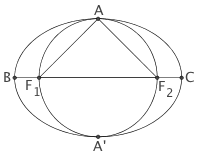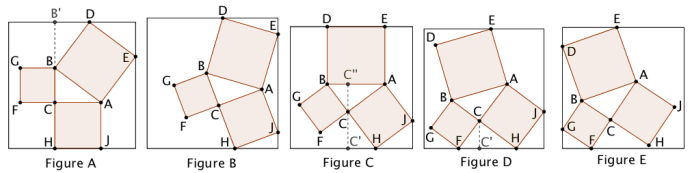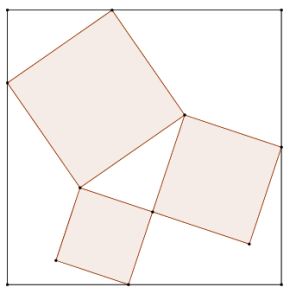## ________________

“Circles, squares, ellipses,” mutters Coach Newton. “We’ve focused on all of those, but we need to spend a week on something more basic: angles!”

## ________________### The Second Bisection

Circle a with center A intersects line s at points B and D. Line u is the perpendicular bisector of segment AB. Line u intersects circle a at point G on the same side of line s as A. Line v is the angle bisector of angle DAG.

What is the acute angle between lines s and v?

### The Third Circle

Circle a with center A intersects line s at points B and D. Circle b with center B goes through A and intersects a at point C on the opposite side of s from A. Circle b also intersects s inside a at the point E. Circle c with center C goes through E and intersects a outside of b at point F. Line t goes through F and A.

What is the acute angle between lines s and t?

## Solutions to week 71

Eye Like It.Sam’s circle and ellipse are shown in the diagram, with foci F1 and F2, points of tangency A and A‘, and major axis BC. The ellipse is defined as the collection of all points whose sum of distances to the two foci is constant. But that constant sum turns out to be equal to the length of the major axis: F1C = BF2 by symmetry, so BC = BF1 + F1C = BF1 + BF2. So we only need to compute the sum of the distances to the foci from any point on the ellipse to find the length of the major axis. It’s easiest to find this sum at A, since AF1F2 forms an isosceles right triangle with hypotenuse equal to two. Therefore, each of AF1 and AF2 has length √2. Summing these lengths, the major axis of the ellipse is 2√2.

Pythagorean Poster. Starting with a “standard” orientation of the Pythagorean diagram with respect to the horizontal and vertical axes, the figures below show the five successive counterclockwise rotations of the diagram such that at least two points of the diagram touch one side of the outer square representing the boundaries of the poster.The next possible such rotation would just be a 90-degree rotation of Figure A, with one side of each of the two smaller squares against edges of the poster, and thereafter all of the configurations repeat, rotated by a multiple of 90 degrees. So one of these five must occupy the smallest square. By inspection, each of the figures is taller than it is wide, so we just want the smallest height of any of these figures. Furthermore, to get from Figure B to Figure C, the diagram is pivoting around the lowest point H so that the highest point D is moving lower, so the height of Figure B is definitely greater than that of Figure C. Therefore, Figure B cannot be the best orientation. Similarly, to go from Figure E to Figure D, the diagram is just pivoting around the lowest point F so that the highest point E moves lower, so Figure E cannot be the best orientation.

So to finish the problem, we just need to compute the heights of Figures A, C, and D; whichever is smallest will be the desired answer. The height of Figure A is easy to compute. HC is 20 cm, CB is 15 cm, and BB‘ is the long leg of a 3-4-5 triangle with hypotenuse 25 cm, or 20 cm. So the height of Figure A is 20 + 15 + 20 = 55 cm. The height of Figure C is only slightly more challenging. CC is the long leg of a 3-4-5 triangle with hypotenuse 20 cm, or 16 cm; CC” is the short leg of a 3-4-5 triangle with hypotenuse 20 cm, or 12 cm; and BD is 25 cm. So the height of Figure C is 16 + 12 + 25 = 53 cm.

That leaves just the height of Figure D to compute; our strategy will be to calculate the coordinates of a series of points, culminating in the highest point E. Take point C‘ to be the origin. Since CC‘ and HC‘ are the short and long legs of a 3-4-5 triangle with hypotenuse 20 cm, respectively, the coordinates of C are (0,12) and of H are (16, 0). Since moving from H to C consists of moving 16 units left and 12 units up, and AC is perpendicular to and the same length as CH, moving from C to A consists of moving 16 units up and 12 units right. So the coordinates of point A are (12,28). Similarly, FC‘ is the short leg of a 3-4-5 triangle with hypotenuse 15, and so point F has coordinates (-9,0). Therefore, moving from F to C consists of moving 9 units right and 12 units up, so moving from C to B consists of moving 9 units up and 12 units left. Hence, the coordinates of point B are (-12, 21). Finally, moving from B to A consists of moving 24 units right and 7 units up, so moving from point A to E consists of moving 24 units up and 7 units left. Thus, the coordinates of point E are (5,52).

This calculation establishes that Figure D has the least height, and so Jules needs a square poster at least 52 cm on a side to fit the diagram (in the orientation shown or any of its rotations by a multiple of 90 degrees.)

Coach Newton provides further detail:You know, some of my team members may have been a little hasty in the advice they gave to Jules. Not that it changes the answer, but conceptually speaking, in a situation like this, there is one other type of configuration at which the minimum square size might occur: when at least one point of the diagram touches each side of the square. And in the case of this 3-4-5 Pythagorean diagram, there is one configuration like that, as shown. (It’s the only one, up to 90 degree rotations.) Now, when the diagram is in this position, the square needs to be significantly bigger — almost 54 centimeters on a side — so what the team said was strictly speaking true, but they were being a little cocky when they implied that you didn’t even need to look for configurations like this one. In fact, if you tried to solve the exact same problem for the 5-12-13 right triangle, you would find that the minimum size square would correspond to an orientation in which one point of the diagram touches each side of the square (and no side has two points touching it).

## Recent Weeks

Links to all of the puzzles and solutions are on the Complete Varsity Math page.

Come back next week for answers and more puzzles.

[asciimathsf]